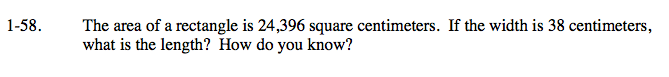### Home > CAAC > Chapter 1 > Lesson 1.2.2 > Problem1-58

1-58.The formula for the area of a rectangle is at right.

Fill in the known values.

Solve.

$\text{Area} = \text{Length} \cdot \text{Width}$

$24396\text{ cm}^2 = \text{ Length }\cdot 38\text{ cm}$

642 cm = Length